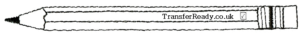###### Function machines are a really useful visual metaphor for equations.

An equation, such as y = 2x + 3 can be represented by the rule of a function machine. Understanding equations is essential for GCSE Maths so younger students are introduced to function machines at the end of KS2 – and they will appear on the AQE and GL transfer tests.

So, lets look at an example…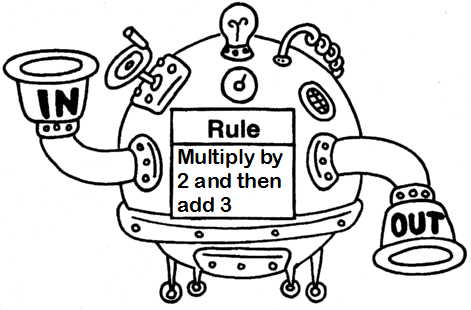This (rather fancy) function machine will take whatever number is ‘input’ (say, 3 for example) and firstly, double the number (3 → 6) and then add three (6 → 9). The resultant number is then ‘output’ from the machine (9 in our example).

An important note is that you must follow the order of the rule – in the example above you must multiply by two before adding on three. If you did it the other way, you would get the wrong answer.

So, how would this appear in the transfer test? Well, it probably wouldn’t have a large Victorian machine in the question, just a series of boxes. Consider the following…

######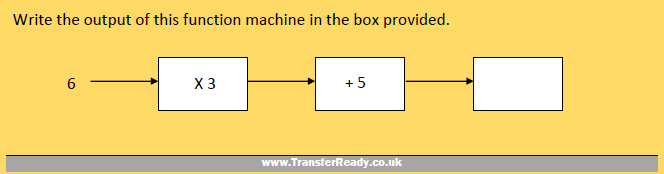In this Step1 question, you get the marks by filling in the correct number in the box.

[hint: the correct answer is 23]

The question can be made more challenging by giving the output number, and asking for the corresponding input number. In this question, you must work backwards through the machine doing the reverse of all the instructions. Consider example 2…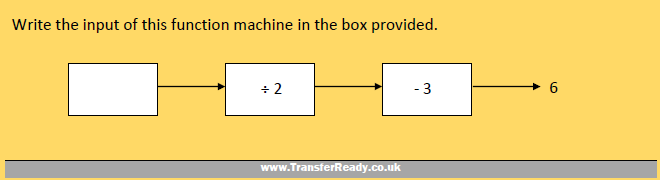To tackle this, you should write the reverse operations beneath the boxes.

The reverse of ‘dividing by 2’ is ‘multiplying by 2’ and the reverse of ‘subtracting 3’ is ‘adding 3’…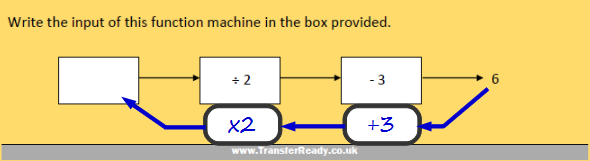Next, write the ‘answers’ of the reverse operations. Do them one at a time, starting at the end and moving back…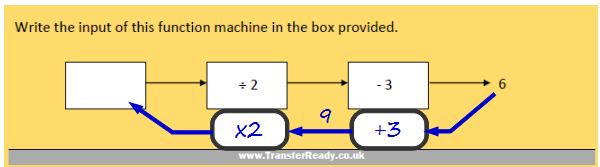Keep filling them in until you run out of reverse operations. Then don’t forget to write your answer in the answer space.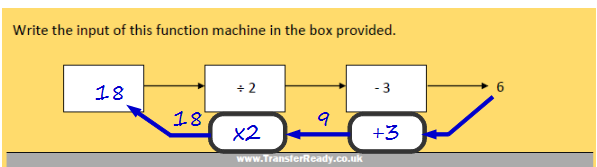After you write your answer in the box, remember to check your answer by running it through the machine in the correct direction. [18 ÷ 2 = 9 | 9 – 3 = 6]

Try to encourage your child to not do these questions by a ‘guess-the-number’ method. The answer in the higher grade questions may be a mixed number (such as 2½) which is nearly impossible to guess.